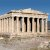Games
Problems
Go Pro!

# The Golden Ratio

Reference > MathematicsThe Golden Ratio -- decimal representation, exact value, algebraic representation, and some history.The Golden Ratio shows up in nature -- the number of spirals on many plants, the shape of a seashell, and moreThe Golden Ratio appears in geometry and trigonometry, in a 72-72-36 triangle.The Golden Ratio can be written as a continued fraction, as shown with proof on this page.The Golden Ratio can be written as a continued radical, as shown with proof on this page.The Golden Ratio is the limit of the ratios of successive terms of a Fibonacci Sequence.If a Fibonacci Sequence is also a geometric sequence, the ratio of terms is the Golden Ratio.When you combine The Golden Ratio with some numerology, you get an amusing result: The Mark of the Beast!Like us on Facebook to get updates about new resources